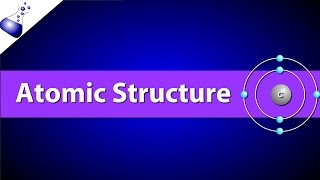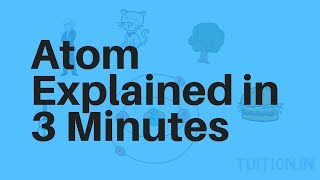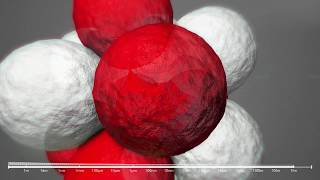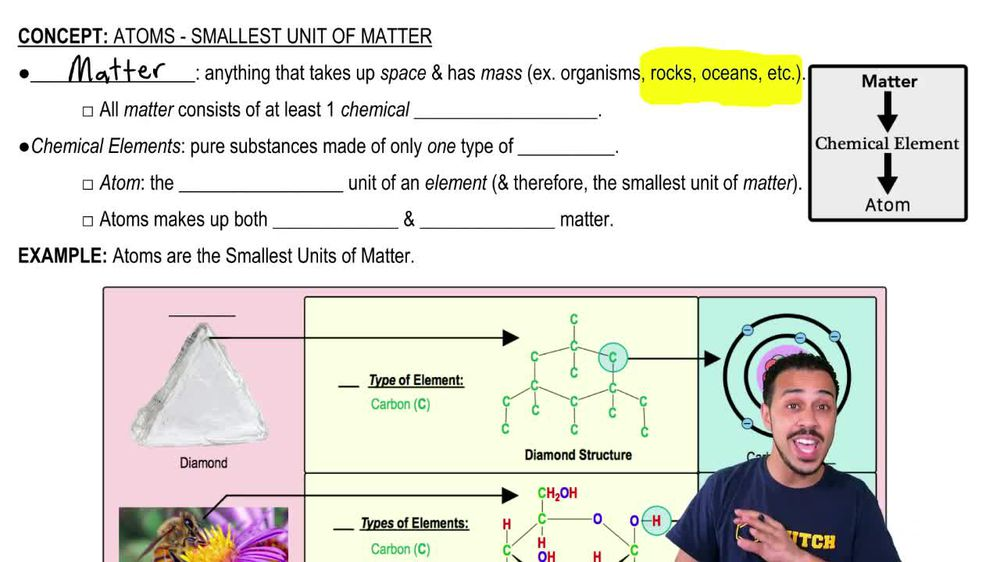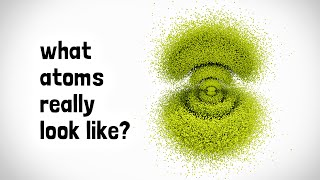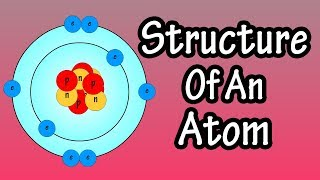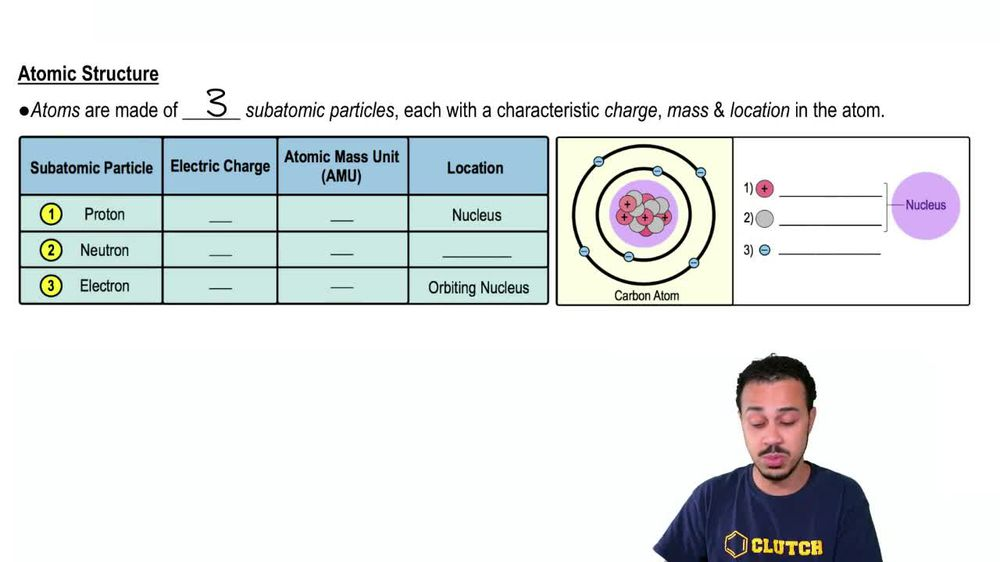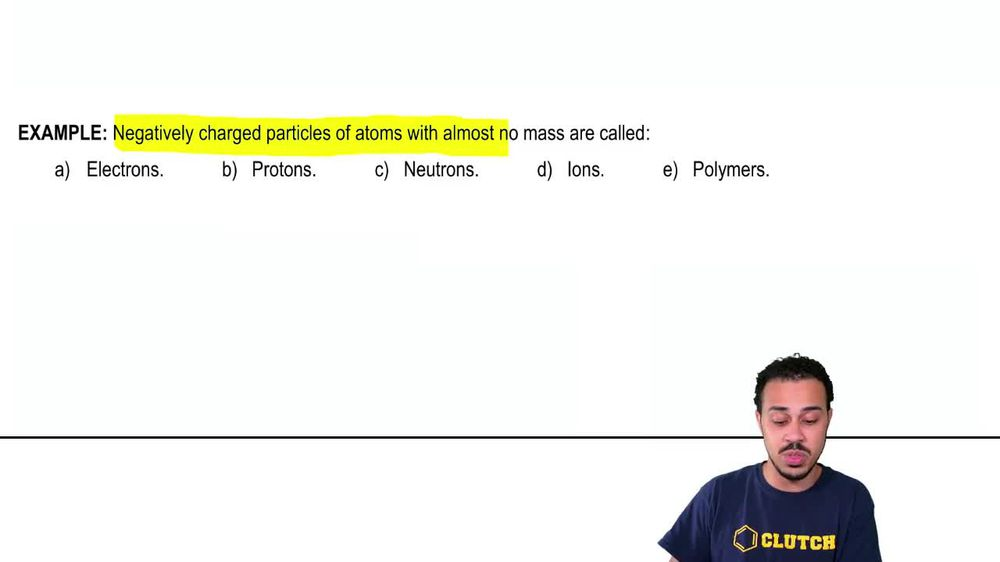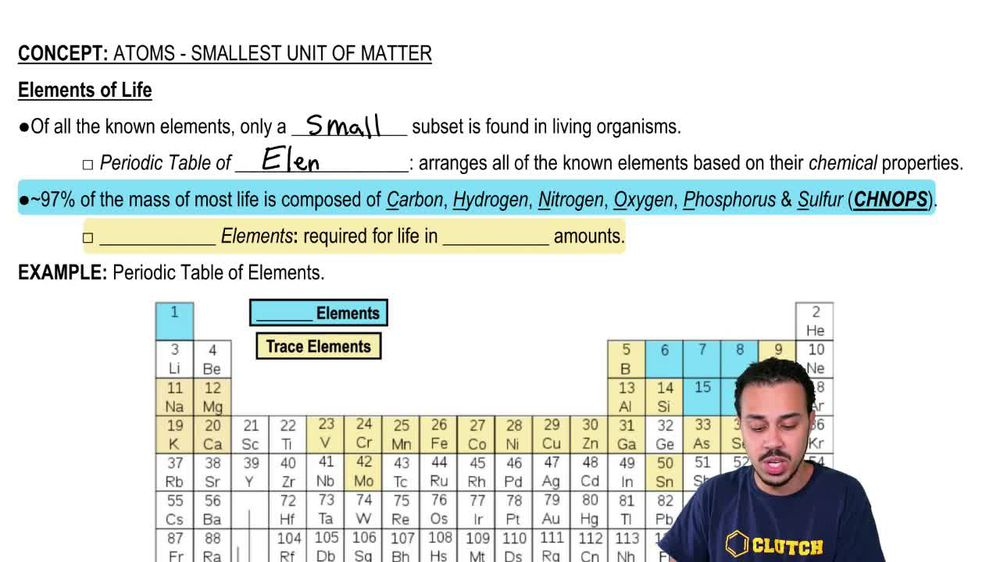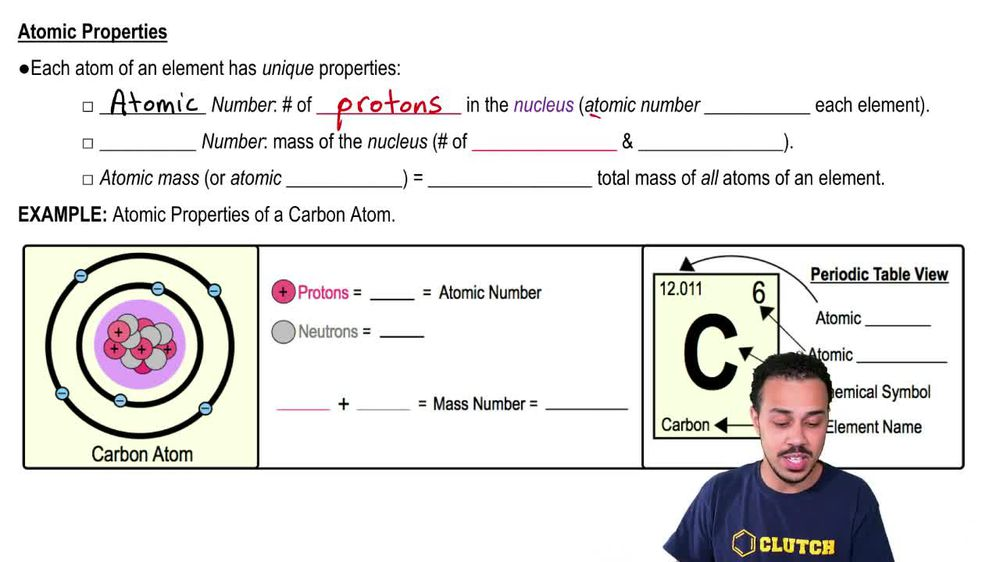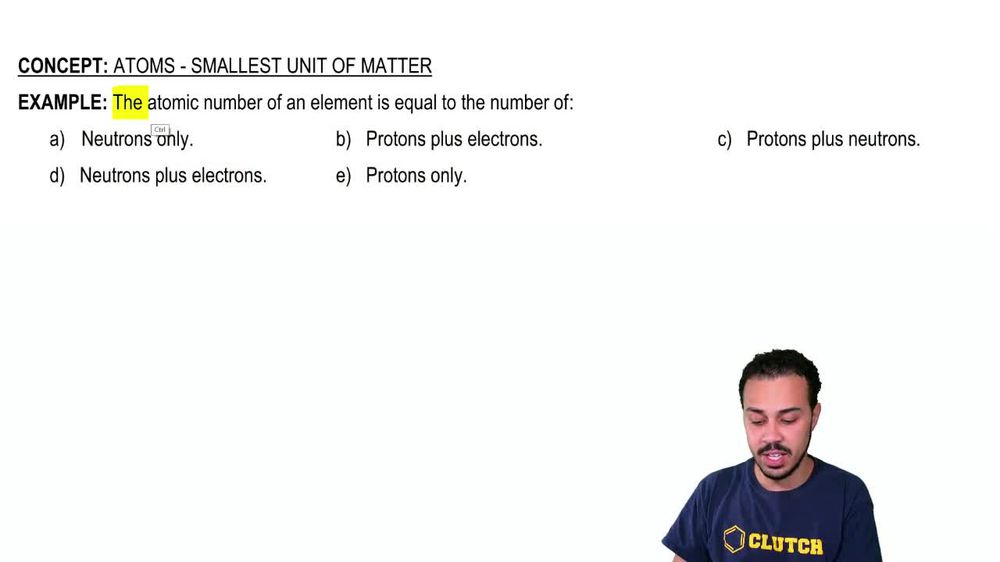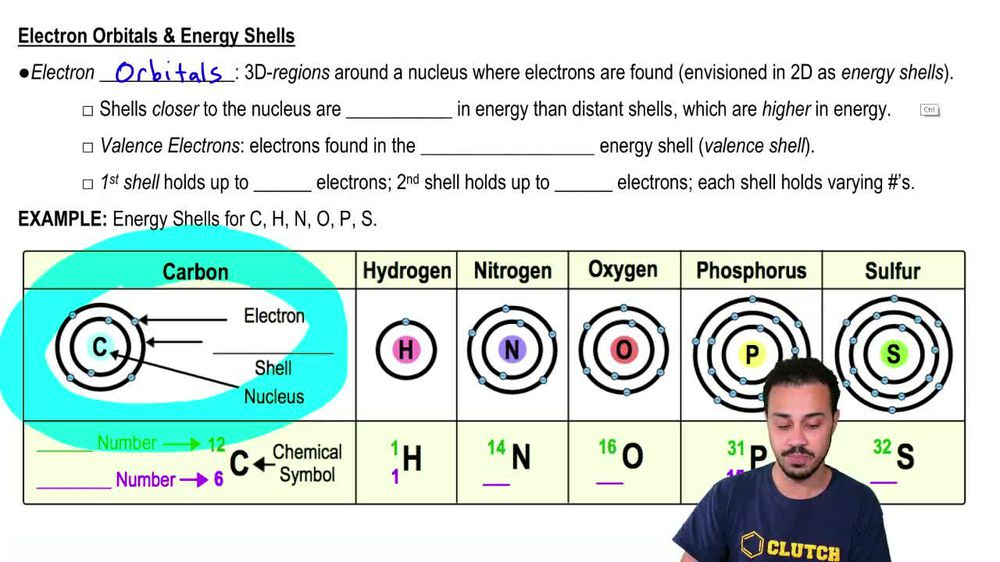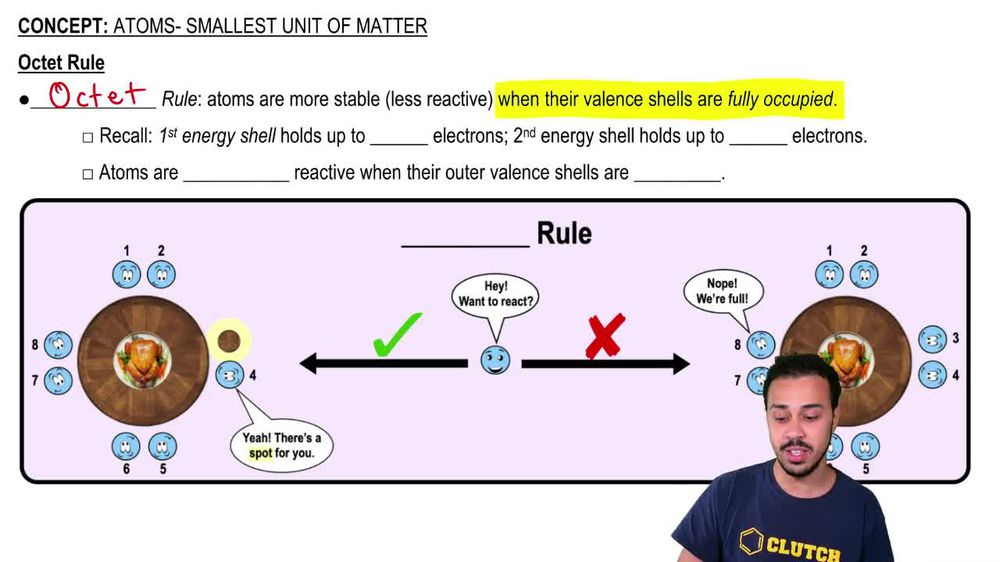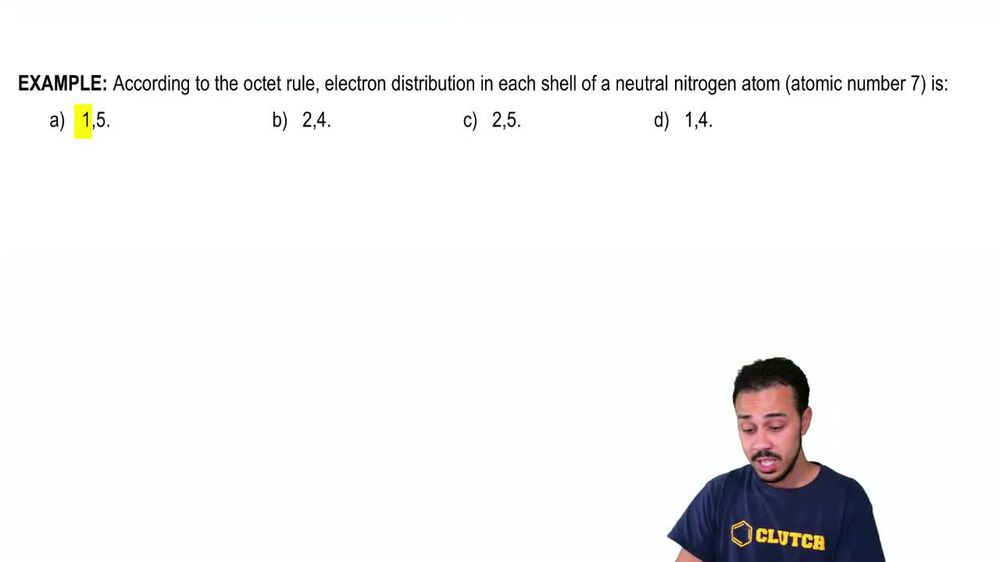Start typing, then use the up and down arrows to select an option from the list.
1. 2. Chemistry2. Atoms- Smallest Unit of Matter# Atoms- Smallest Unit of Matter Example 2

by Jason Amores Sumpter
364 views
6
6
all right. So here we have an example problem that once it's the complete the sentence here, using one of these five potential answer options down below. And it says that the atomic number of an element is equal to the number of neutrons, Onley, neutrons, plus electrons, protons plus electrons, protons, Onley or protons, plus neutrons. Now, of course, we know from our last lesson video that the atomic number is going to be exactly equal to the number of protons Onley that air found in the nucleus. And so this here is our atomic number, and it is the correct answer to this example. Problem. Now, of course, protons plus neutrons might sound familiar, but that's because this is referring to the mass number or the mass of the nucleus of an atom. But it's not the same thing as the atomic number, so that's why it's not correct. The protons plus electrons are going to dictate the net charge of the atom because, remember, protons are positively charged and electrons are negatively charged. And so the balance of protons and electrons, which are opposite Lee charged, is going to dictate the overall net charge of the atom But once again, Option B here is not going to be the atomic number. And then neutrons only And neutrons plus electrons. They once again are not gonna be the atomic number. So that concludes this example problem. And I'll see you in our next video.